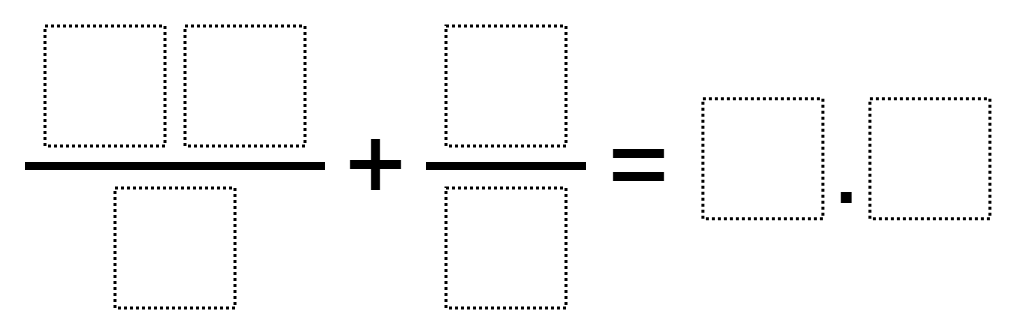Home > Grade 5 > Sum of Fractions Closest to 10

# Sum of Fractions Closest to 10

Directions: Using the digits 1 through 9, at most one each time, fill in the boxes to make the statement true.### Hint

How do we add fractions? If we want to get the closest to 10, what does that mean for each of the fractions?

### Answer

So far, this is the closest answer: 1/2 + 46/5 = 9.7

Source: Nanette Johnson, based on Giselle Garcia’s problem

## Multiplying Decimals to Make a Whole Number Product

Directions: Using the digits 1 to 9 at most one time each, fill in the …

### 4 comments

1.I had a student come up with 9.8 as a solution:
34/5 + 6/2 = 9.8

•How??? Where should a kid start? My child needs to show the steps to solve this problem. Where do we start?

•Since the answer contains a whole number and partial number (__.__), you can plug digits into the improper fraction that would get you a whole number 9, which is as close to 10 as you can get, and then try to plug in other digits that would give you the greatest value of partial number. You have to play with it more than follow steps because of the instruction that digits can only be used once.

2.63/7 + 4/5=9.8
27/3+4/5=9.8